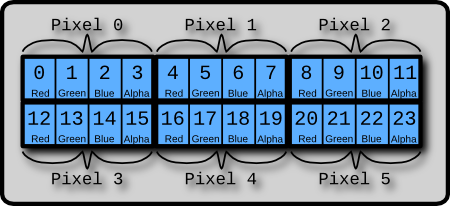悄然无声的 Blog 不是我不明白，这世界变化快！

 提示：建议使用 Chrome 浏览器或者 Firefox 浏览器阅读本文，否则文中的样例程序可能无法看到运行效果。   一、理解颜色 我们在电脑屏幕上可以看到色彩斑斓的图像，其实这些图像都是由一个个像素点组成的。那么像素是什么？颜色又是什么呢？（如果您提出这两个问题，您一定是个热爱思考的人）一个像素其实对应着内存中的一组连续的二进制位，由于是二进制位，每个位上的取值当然只能是 0 或者 1 了！这样，这组连续的二进制位就可以由 0 ，1 排列组合出很多种情况，而每一种排列组合就决定了这个像素的一种颜色。先看看下面这幅图ImageData对象用来保存图像像素值，它有 width、height和 data 三个属性，其中 data 属性就是一个连续数组，图像的所有像素值其实是保存在 data 里面的。

data 属性保存像素值的方法和我们在前面图片中看到的一模一样：

imageData.data[index*4 +0]

imageData.data[index*4 +1]

imageData.data[index*4 +2]

imageData.data[index*4 +3]

createImageData(width, height)：在内存中创建一个指定大小的 ImageData 对象（即像素数组），对象中的像素点都是黑色透明的，即rgba(0,0,0,0)

getImageData(x, y, width, height)：返回一个 ImageData 对象，这个 IamgeData 对象中包含了指定区域的像素数组

putImageData(data, x, y)：将 ImageData 对象绘制到屏幕的指定区域上

1、将一幅图片绘制到一个 canvas 元素上，为了不引发安全错误(Security_ERR:DOM EXCEPTION 18)，我用的是我博客顶部的横幅背景图片。你要运行这个例子，可能需要改成自己的图片

2、有四个滑动条，分别代表 GRBA 四个分量

3、拖动滑动条，图像中对应的颜色分量就会增加或者减少

4、如果图像变成透明，就会显示 canvas 元素的背景，我把这个背景设置成了我的头像，呵呵。简单的图像处理
<canvas id="test1" width="507" height="348" style="background-image:url(http://images.cnblogs.com/cnblogs_com/myqiao/262115/r_2204793492575248335.jpg)">你的浏览器不支持 &lt;canvas&gt;标签，请使用 Chrome 浏览器 或者 FireFox 浏览器</canvas>

<script type="text/javascript">

//获取上下文对象
var canvas = document.getElementById("test1");

var ctx = canvas.getContext("2d");

//画布的宽度和长度
var width = parseInt(canvas.getAttribute("width"));

var height = parseInt(canvas.getAttribute("height"));

//装入图像
var image = new Image();
//顶部背景图片
image.src = "/skins/Valentine/images/banner2.gif";

//用来保存像素数组的变量
var imageData=null;

// 将图片画到画布上
ctx.drawImage(image, 00);

//取图像的像素数组
imageData = ctx.getImageData(00, width, height);
}

function colorChange(event,offset){

for (var y = 0; y < imageData.height; y++) {

for (x = 0;x < imageData.width; x++) {

//index 为当前要处理的像素编号
var index = y * imageData.width + x;

//一个像素占四个字节，即 p 为当前指针的位置
var p = index * 4;

//改变当前像素 offset 颜色分量的数值，offset 取值为0-3
var color = imageData.data[p + offset] * event.target.value / 50

// 颜色值限定在[0..255]
color = Math.min(255, color);

//将改变后的颜色值存回数组
imageData.data[p + offset]=color
}
}

//输出到屏幕
ctx.putImageData(imageData, 00);
}
</script>随机颜色的点
<canvas id="test2" width="300" height="300" style=" background-color: black">你的浏览器不支持 &lt;canvas&gt;标签，请使用 Chrome 浏览器 或者 FireFox 浏览器</canvas>
<input type="button" value="画随机点" onclick="interval=setInterval(randomPixel,1);" />
<input type="button" value="停止" onclick="clearInterval(interval);"/>
<input type="button" value="清除" onclick="clearCanvas();"/>
<script type="text/javascript">

//获取上下文对象
var canvas = document.getElementById("test2");

var ctx = canvas.getContext("2d");

//画布的宽度和长度
var width = parseInt(canvas.getAttribute("width"));

var height = parseInt(canvas.getAttribute("height"));

var imageData = ctx.createImageData(width, height);

function randomPixel(){

var x= parseInt(Math.random()*width);

var y= parseInt(Math.random()*height);

var index = y * width + x;

var p = index * 4;

imageData.data[p
+ 0= parseInt(Math.random() * 256);
imageData.data[p
+ 1= parseInt(Math.random() * 256);
imageData.data[p
+ 2= parseInt(Math.random() * 256);
imageData.data[p
+ 3=parseInt(Math.random() * 256);
ctx.putImageData(imageData,
0,0);
}

function clearCanvas(){
ctx.clearRect(
0,0,width,height);
imageData
= ctx.createImageData(width, height);
}
</script>

HTML5边玩边学（1）：画布

HTML5边玩边学（2）：基础绘图

//==================================================

posted on 2010-09-18 22:40  左洸  阅读(...)  评论(...编辑  收藏